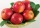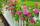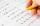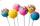# Greatest common divisor (GCD) - math word problems

#### Number of problems found: 611st blade 2,5 m, 2nd blade. .1.75 m. How many same long pieces of this two blades can be do the biggest? How long is one piece?
• Children's homeThe children's home received a gift to Nicholas of 54 oranges, 81 chocolate figurines, and 135 apples. Every child received the same gift and nothing was left. a) How many packages could be prepared? b) what did the children find in the package?
• Largest squaresHow many of the largest square sheets did the plumber cut the honeycomb from 16 dm and 96 dm?
• The classroomThe classroom is 9 meters long. The width of the classroom is smaller and can be passed in equally long steps of 55 CM or 70 CM. Determine the width of the classroom.
• TilesHall has dimensions 325 &time; 170 dm. What is the largest size of square tiles that can be entire hall tiled and how many we need them?
• Diofant equationIn the set of integers (Z) solve the equation: ? Write result with integer parameter ? (parameter t = ...-2,-1,0,1,2,3... if equation has infinitely many solutions)
• Square tilesThe room has dimensions of 12 meters and 5.6 meters. Determine the number of square tiles and their largest dimension to exactly cover the floor.
• Wide fieldThe field is 203 meters wide 319 meter long, what is the greater length of the rope by which length and width can be measured and find the exact number of time.
• Around the flowerbedAround a rectangular flowerbed with dimensions 5.25 m and 3.5 m are to be planted roses equally spaced so that the roses found in every corner of the flowerbed and consumed them as little as possible. a) At what distance are planted roses? b) How many ros
• Cutting paperDivide a rectangular paper with dimensions 220mm and 308mm into squares of the same size so that they are as large as possible. Specify the length of the side of the square.
• 9.A9.A to attend more than 20 students but fewer than 40 students. A third of the pupils wrote a math test to mark 1, the sixth to mark 2, the ninth to mark 3. No one gets mark 4. How many students of class 9.A wrote a test to mark 5?
• Paper squaresThe paper rectangle measuring 69 cm and 46 cm should be cut into as many squares as possible. Calculate the lengths of squares and their number.
• Four poplarsFour poplars are growing along the way. The distances between them are 35 m, 14 m, and 91 m. At least how many poplars need to be dropped to create the same spacing between the trees? How many meters will it be?
• Three friendsThree friends had balls in ratio 2: 7: 4 at the start of the game. Could they have the same number of balls at the end of the game? Write 0, if not, or write the minimum number of balls they had together.
• PegsFrom two sticks 240 cm and 210 cm long, it is necessary to cut the longest possible pegs for flowers so that no residues remain. How many pegs will it be?
• Trees in alleyThere are four trees in the alley between which the distances are 35m, 15m and 95m. Trees must be laid in the spaces so that the distance is the same and the maximum. How many trees will they put in and what will be the distance between them?
• The woodenThe wooden block measures 12 cm, 24 cm, and 30 cm. Peter wants to cut it into several identical cubes. At least how many cubes can he get?
• PexesoDrawing paper has dimensions of 60cm and 840mm. Pupils have to divide it into squares so that they can make a pexeso. What dimension must squares have if their side is to be larger than 3cm and less than 10cm?
• Identical cubesFrom the smallest number of identical cubes whose edge length is expressed by a natural number, can we build a block with dimensions 12dm x 16dm x 20dm?
• BirthdateJane on birthday brought 30 lollipops and 24 chewing gum for their friends. How many friends has, if everyone receives the same number of lollipops and chewing gums? How much chewing gum and lollipops got any friend?

Do you have an interesting mathematical word problem that you can't solve it? Submit a math problem, and we can try to solve it.

We will send a solution to your e-mail address. Solved examples are also published here. Please enter the e-mail correctly and check whether you don't have a full mailbox.

Please do not submit problems from current active competitions such as Mathematical Olympiad, correspondence seminars etc...

Do you want to calculate greatest common divisor two or more numbers?